Question

# Two isolated conducting spheres are separated by a large distance. Sphere 1 has a radius of...

Two isolated conducting spheres are separated by a large distance. Sphere 1 has a radius of 20 cm and an initial charge 30 nC while Sphere 2 has a radius of 60 cm and an initial charge 70 nC. A very thin copper wire is now connected to the spheres to allow charge to ﬂow between them. How much charge will be transferred from Sphere 2 to Sphere 1? (Note that the charge transferred can be positive, negative or zero.)

Dear student,

Find this solution, and RATE IT ,If you find it is helpful .your rating is very important to me.If any incorrectness ,kindly let me know I will rectify them soon.

Thanks for asking ..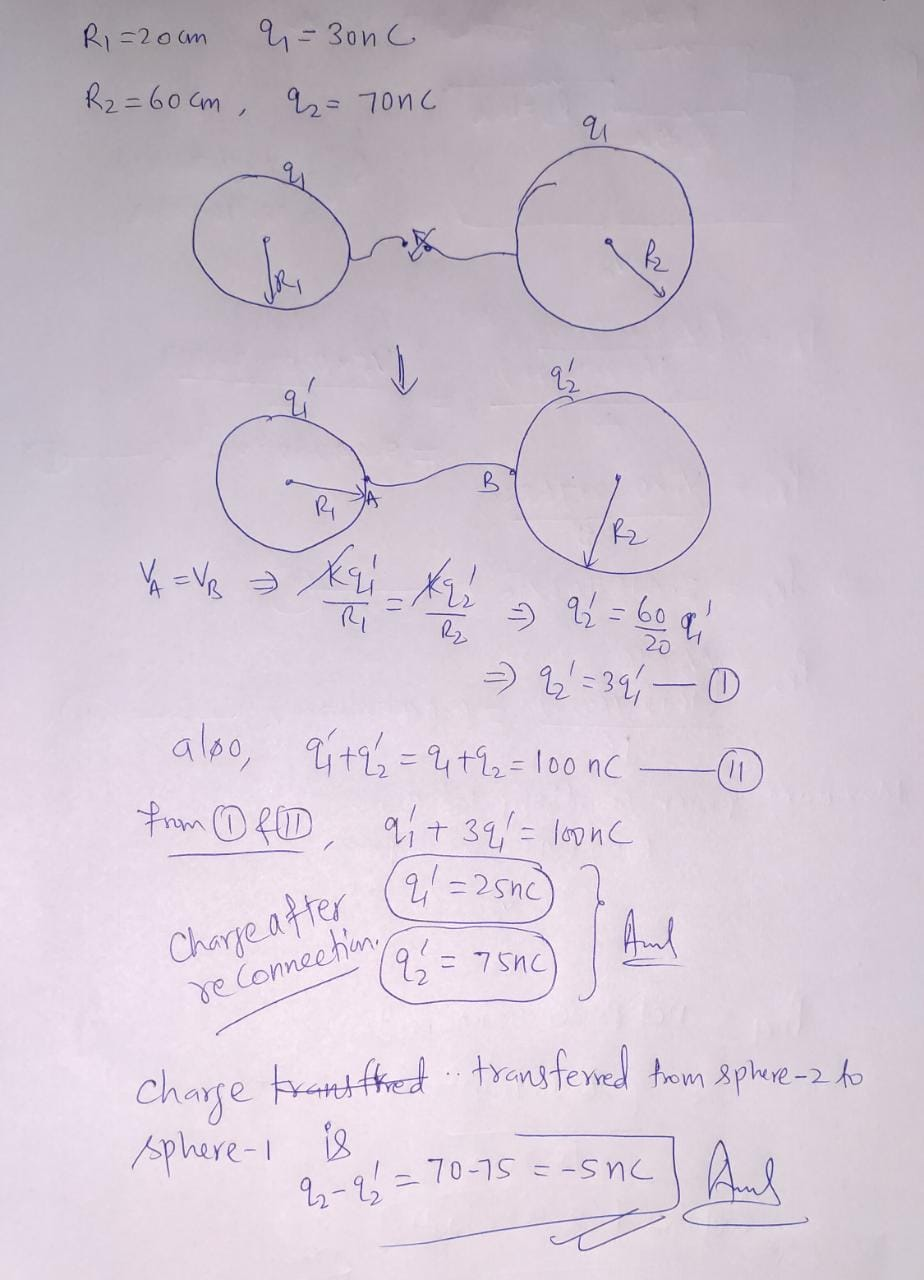#### Earn Coins

Coins can be redeemed for fabulous gifts.

Similar Homework Help Questions
• ### Two spherical conductors are separated by a distance much larger than either of their radii. Sphere...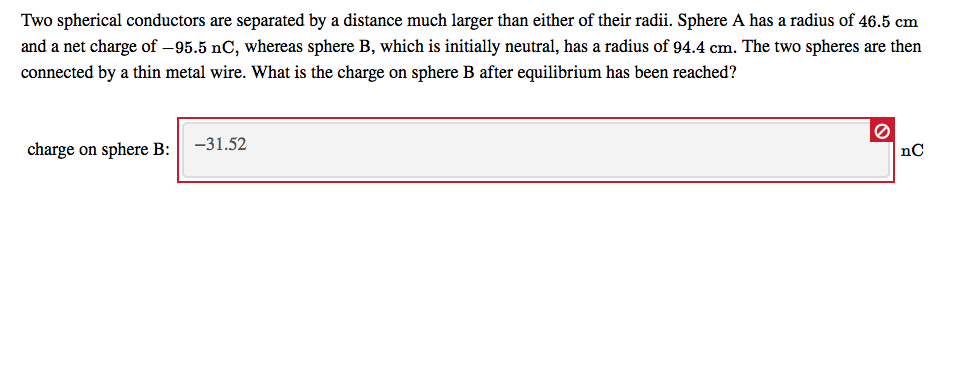Two spherical conductors are separated by a distance much larger than either of their radii. Sphere A has a radius of 46.5 cm and a net charge of -95.5 nC, whereas sphere B, which is initially neutral, has a radius of 94.4 cm. The two spheres are then connected by a thin metal wire. What is the charge on sphere B after equilibrium has been reached? charge on sphere B: -31.52 nc

• ### two conducting spheres

Charge is placed on two conducting spheres that are very far apart and connected by a long thin wire. The radius of the smaller sphere is 5 cm and that of the largersphere is 12 cm. The electric field at the surface of the larger sphere is 630 kV/m. Find the surface charge density on each sphere.

• ### Charge is placed on two conducting spheres that are very far apart and connected by a...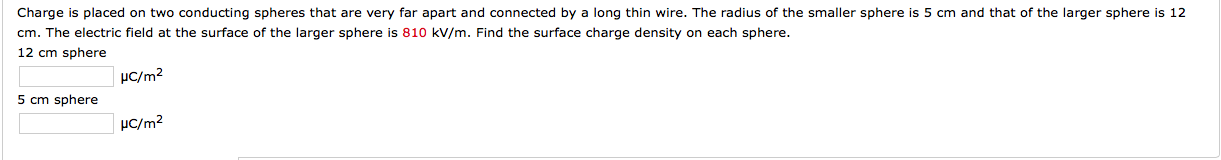Charge is placed on two conducting spheres that are very far apart and connected by a long thin wire. The radius of the smaller sphere is 5 cm and that of the larger sphere is 12 cm. The electric field at the surface of the larger sphere is 810 kV/m. Find the surface charge density on each sphere.

• ### Identical isolated conducting spheres 1 and 2 have equal charges and are separated by a distance that is large compared with their diameters

Identical isolated conducting spheres 1 and 2 have equal charges and are separated by a distance that is large compared with their diameters. The electrostatic force acting on sphere 2 due to sphere 1 is F. Suppose now that a third identical sphere 3, having an insulating handle and initially neutral, is touched first to sphere 1, then to sphere 2, and finally removed. The electrostatic force that now acts on sphere 2 has magnitude F'. What is the ratio...

• ### 4. Two conducting spheres are far apart. The smaller sphere has radius R and carries a...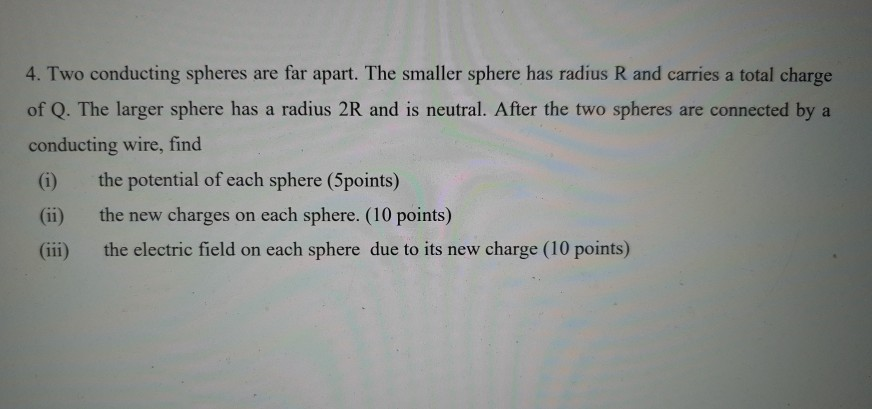4. Two conducting spheres are far apart. The smaller sphere has radius R and carries a total charge of Q. The larger sphere has a radius 2R and is neutral. After the two spheres are connected by a conducting wire, find the potential of each sphere (5points) the new charges on each sphere. (10 points) (iii) the electric field on each sphere due to its new charge (10 points)

• ### 4. Two conducting spheres are far apart. The smaller sphere has radius R and carries a...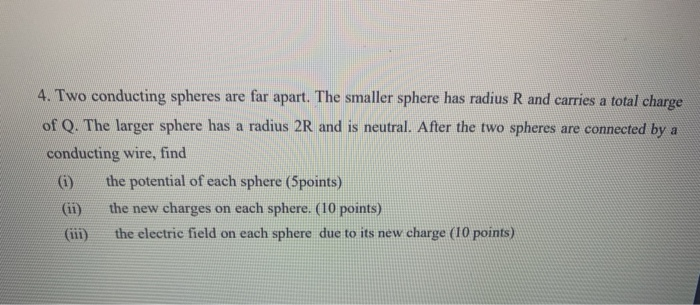4. Two conducting spheres are far apart. The smaller sphere has radius R and carries a total charge of Q. The larger sphere has a radius 2R and is neutral. After the two spheres are connected by a conducting wire, find (1) the potential of each sphere (5points) the new charges on each sphere. (10 points) the electric field on each sphere due to its new charge (10 points)

• ### 4. Two conducting spheres are far apart. The smaller sphere has radius R and carries a...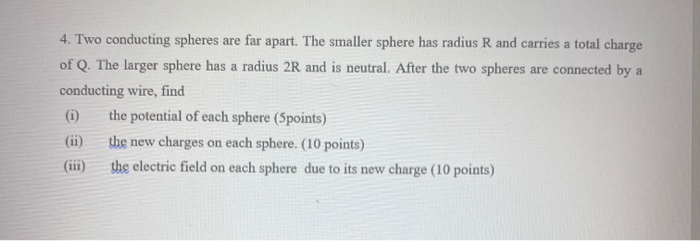4. Two conducting spheres are far apart. The smaller sphere has radius R and carries a total charge of Q. The larger sphere has a radius 2R and is neutral. After the two spheres are connected by a conducting wire, find (1) the potential of each sphere (Spoints) the new charges on each sphere. (10 points) (iii) the electric field on each sphere due to its new charge (10 points)

• ### 4. Two conducting spheres are far apart. The smaller sphere has radius R and carries a...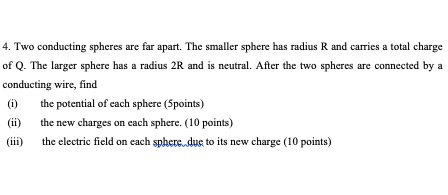4. Two conducting spheres are far apart. The smaller sphere has radius R and carries a total charge of Q. The larger sphere has a radius 2R and is neutral. After the two spheres are connected by a conducting wire, find (1) the potential of each sphere (Spoints) the new charges on each sphere. (10 points) (iii) the electric field on each sphere due to its new charge (10 points)

• ### Charge is placed on two conducting spheres that are very far apart and connected by a...

Charge is placed on two conducting spheres that are very far apart and connected by a long thin wire. The radius of the smaller sphere is 5 cm and that of the larger sphere is 12 cm. The electric field at the surface of the larger sphere is 800 kV/m. Find the surface charge density on each sphere. 12 cm sphere in µC/m2.............................. 5 cm sphere in µC/m2...................................